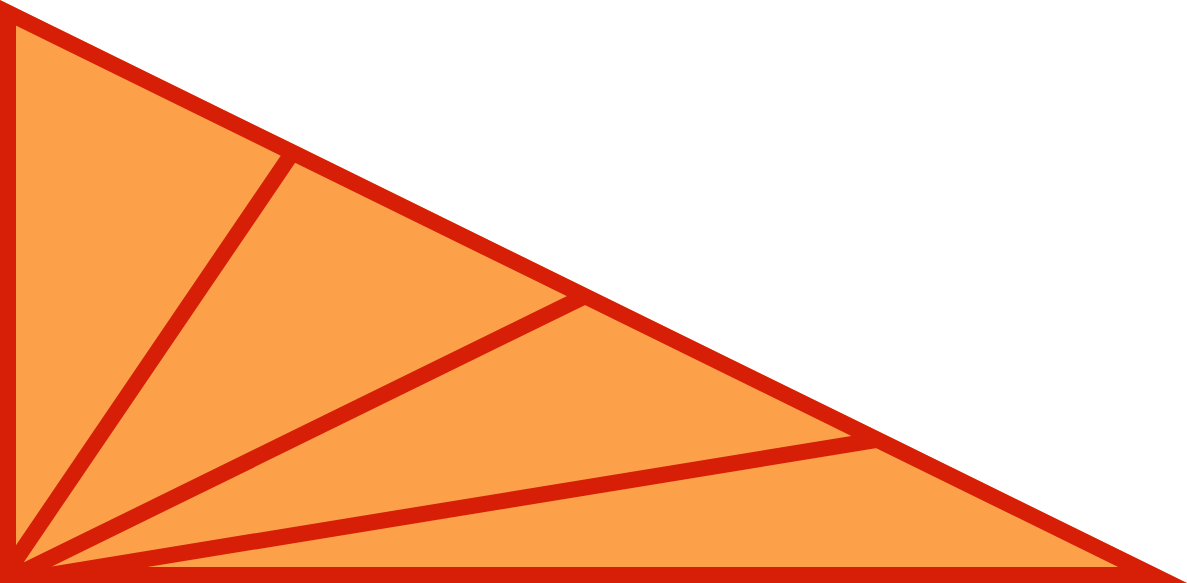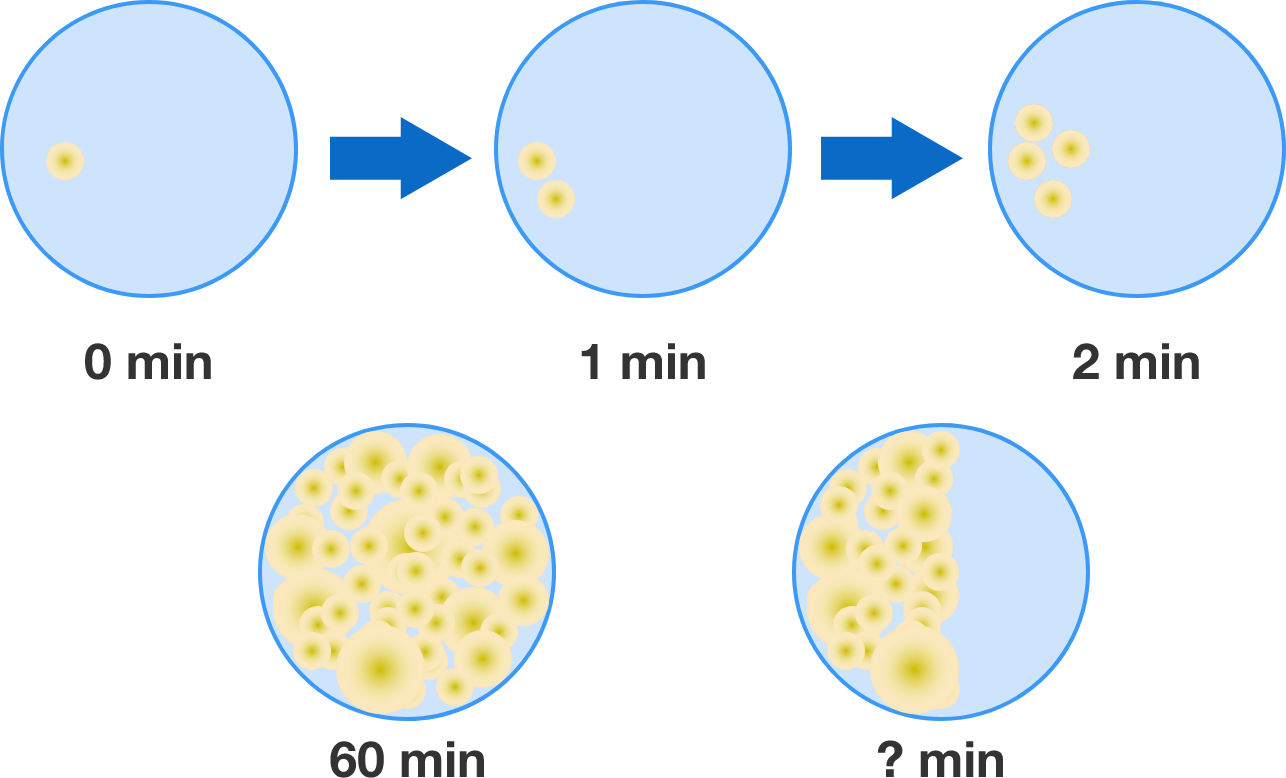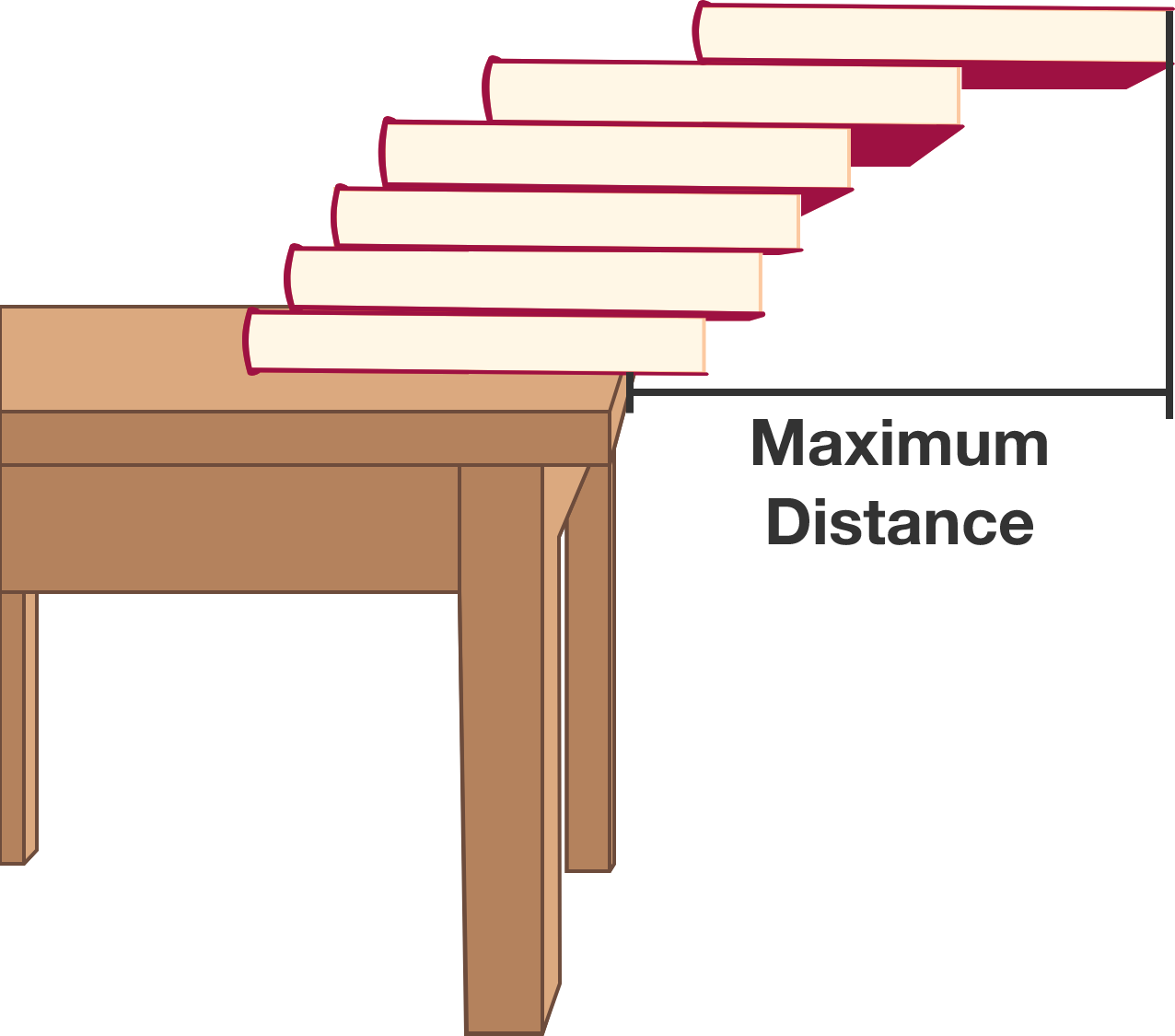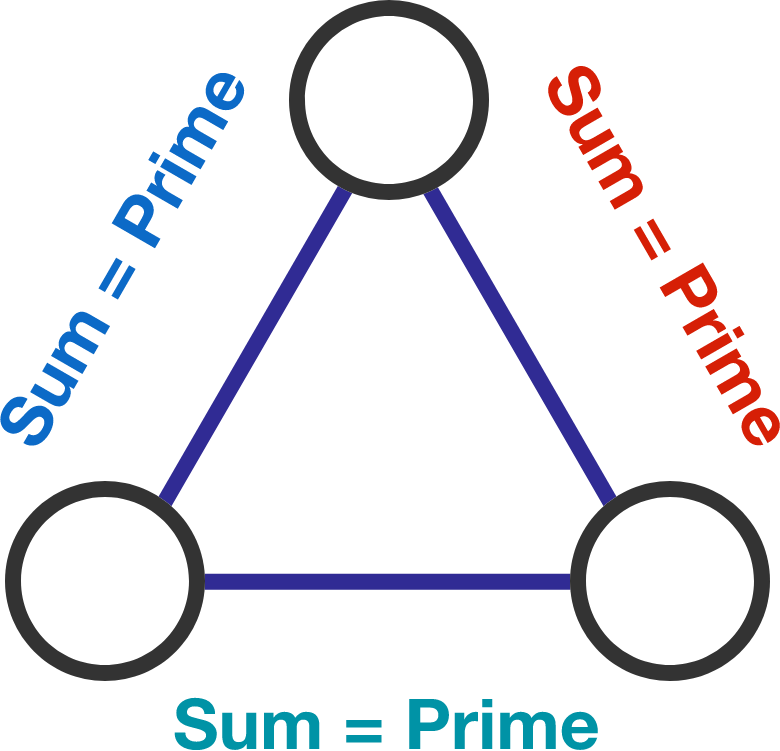# Problems of the Week

Contribute a problem

# 2017-07-31 BasicCount the number of triangles in the picture above.

Hint: The answer is not 4. There's a faster way than simply counting them all!

A certain type of bacteria doubles its coverage area every minute. Exactly after an hour, a Petri dish is totally covered by the bacteria. After how many minutes (from the beginning) did it cover half the dish?Joey has an infinite number of identical books that are rigid and perfectly rectangular. He places them all in a stack on the edge of a table.

What is the maximum distance (parallel to the ground) that the edge of the top book can get from the edge of the table without falling over?

Assume the gravitational field is vertical and uniform.

$\begin{array} {cccc} \large & & \color{#D61F06}C & \color{#3D99F6}A & \color{#20A900}R \\ \large & & & \color{#D61F06}C & \color{#3D99F6}A \\ \large + & & & & \color{#D61F06}C \\ \hline \large & & \color{#3D99F6}A & \color{#20A900}R & \color{#D61F06}C \end{array}$

If $\color{#D61F06}C$, $\color{#3D99F6}A$, and $\color{#20A900}R$ are (not necessarily distinct) non-zero digits, what is the 3-digit final sum $\overline{ {\color{#3D99F6}A} {\color{#20A900}R} {\color{#D61F06}C } }?$

Each circle in the diagram contains a distinct positive integer. Is it possible to fill in the circles such that each pair of adjacent circles adds up to a prime number?×